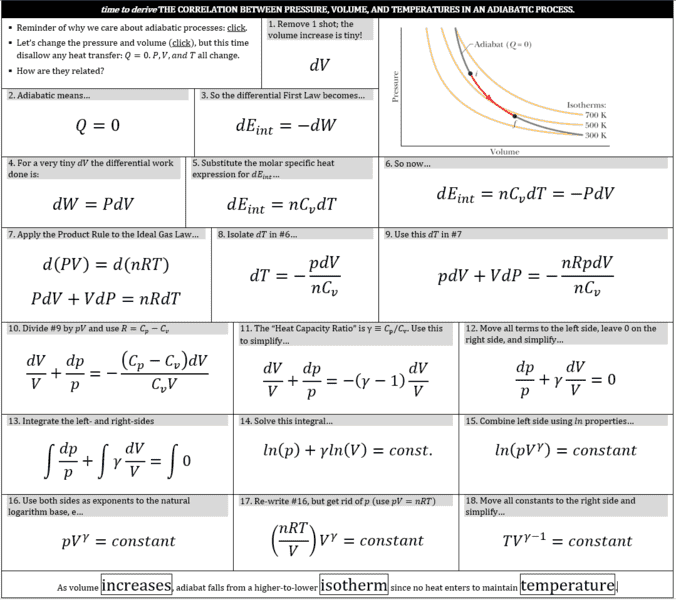# Adiabatic PVT relationships - why Kelvin and not Celsius?

Taulant Sholla

## Homework Statement

Here's a derivation of the adiabatic PVT relationships.

QUESTION: When solving adiabatic PVT homework problems I found that I needed to express temperature in Kelvin, rather than Celsius, to get the right answers. Where in this derivation should this be specified, and how should it be specified - or otherwise how should I know this?

Thank you!## The Attempt at a Solution

Please refer to question above. Thank you!

#### Attachments

Taulant Sholla
My hunch is that the Ideal Gas Law is based on absolute temperature, which is the basis for all of this.

This is a fundamental concept in thermodynamics which I think you should have learned at the very beginning of any course. Temperature in a gas is a measure of the average kinetic energy of the molecules in the gas. This only goes to zero at absolute zero, so all of these expressions in thermodynamics only make sense when using an absolute temperature scale. The zero point of the Celsius scale is arbitrarily set at the freezing point of water. This zero point has no relation to the thermodynamic quantities.

Existoic
Deriving the ideal gas equation is an exciting task in your early physics education.

It should presented as a key idea in either your basic or intermediate level courses at University. Most thermophysics books present it more or less elegantly since the argument is quite straightforward. See for example Gerard Carrington's Basic Thermodynamics Chapter 1. He derives PV=nkT first with what he calls a 'simple-minded' argument and then introduces (in my opinion unnecessary) complexity by completing the same task using integral sums.

The derivation involves the key ideas, (1) that pressure of gas is the intensity of it's molecular strikes against a side of their containing vessel, multiplied by the impacts' average momentum change which is the force they exert on the vessel's side and vice versa; and (2) that temperature is simply a way of measuring the kinetic energy of gas molecules (translational and internal) contained in the gas. The logic of the derivation also introduces k_b, the Boltzmann constant which translates between molecular kinetic energy and the measure of temperature. R - constant is k_b - constant for moles of gas, and the bare k_b is used when working directly with a certain number of gas molecules instead of moles of gas molecules. If you pursue statistical physics, you'll find the idea of temperature is dropped, and energies are talked of in their "natural" units of J and eV, with some occasional reference to what the energies might be when multiplied by k_b and thus expressed as temperatures.

Good luck!

I have a tendency of writing a lot when I think I know something, so any corrections to what I say are much appreciated.